# multiview¶

The multiview module consists of:

• a collection of solvers for 2 to n-view geometry constraints that arise in multiple view geometry,
• a generic framework “Kernel” that can embed these solvers for robust estimation.

First accessible solvers are listed and explained and the “Kernel” concept is documented.

## 2-view solvers (2d-2d correspondences)¶

openMVG provides solver for the following geometric estimation:

• affine,
• homographic,
• fundamental,
• 7 to n pt,
• 8 to n pt (Direct Linear Transform) [HZ].
• essential,

## N-View geometry estimation¶

• Triangulation
• 2 to n view (Direct Linear Transform),
• 2 to n view (Iterated least square).
• Rotation averaging
• Translation averaging

### Homography matrix:¶

The homography matrix maps the relation between two projections of a plane: Figure.

$$H$$ is a (3 x 3) matrix that links coordinates in left and right images with the following relation.

(1)$x'_i = Hx_i$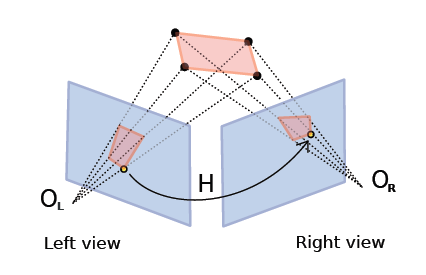The homography matrix and the point to point constraint.

OpenMVG implementation follows the DLT (Direct Linear Transform) explained in [HZ] book: $$H$$ can be estimated from 4 to $$n$$ corresponding points.

### Fundamental matrix:¶

The fundamental matrix is a relation between two images viewing the same scene where those point’s projections are visible in the two images. Given a point correspondence between two views $$(x_i,x'_i)$$:

We obtain the following relation:

$x'^T_i Fx_i = 0$

$$F$$ is the (3 x 3) Fundamental matrix, it puts in relation a point x to a line where belong the projection of the 3D X point. $$l'_i = Fx_i$$ designs the epipolar line on which the point $$x'_i$$ could be. The relation $$x'^T_i Fx_i = 0$$ exists for all corresponding point belonging to a stereo pair.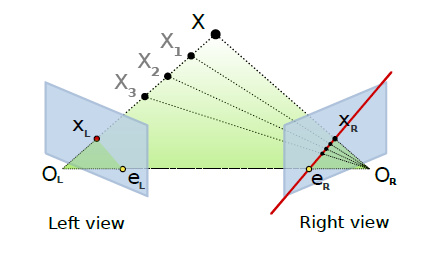The fundamental matrix and the point to line constraint.

The fundamental matrix is sometime called bifocal-tensor, it is a 3 x 3 matrix of rank 2 with 7 degree of freedom. 8 ou 7 correspondences are sufficient to compute the $$F$$ matrix. Implementation follows the DLT (Direct Linear Transform) explained in [HZ] book.

## Relative pose estimation (Essential matrix)¶

Adding intrinsic parameters to the fundamental matrix gives a metric “object” that provides the following relation $$E = K'^T FK$$ , this is the Essential relation explained by Longuet-Higgins in 1981 [Longuet]. This essential matrix links the relative position of the camera to the fundamental matrix relation.

$E = R[t]x = K'^T FK$The essential matrix geometric relation.

## Absolute pose estimation/Camera resection (Pose matrix)¶

Given a list of 3D-2D point correspondences it is possible to compute a camera pose estimation. It consists in estimating the camera parameters of the right camera that minimizes the residual error of the 3D points re-projections, it’s an optimization problem that trying to solve P parameters in order to minimize

$min \sum^n_{i=1} x_i - P(X_i).$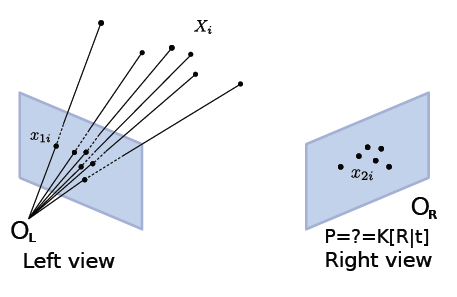Resection/Pose estimation from 3D-2D correspondences.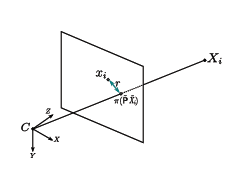Residual error.

openMVG provides 3 different solvers for this problem:

• 6pt Direct Linear Transform [HZ],
• 3pt with intrinsic EPnP [Ke],
• 3pt with intrinsic P3P [Kneip].

### Kernel concept¶

In order to use the solver in a generic robust estimation framework, we use them in conjuction with the Kernel class that allow to link:

• data points,
• the set of correspondences that are used for a robust estimation problem.
• a model solver/estimator,
• a metric to measure data fitting to a putative model.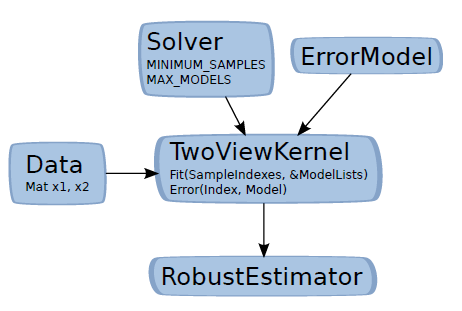The Kernel concept (the two view case).

Solver:
MINIMUM_SAMPLES: The minimal number of point required for the model estimation, MAX_MODELS: The number of models that the minimal solver could return, A Solve function that estimates a model from MINIMUM_SAMPLES to n vector data.
ErrorModel:
An metric function that return the error of a sample data to the provided model.
Kernel:
Embed data (putative), the model estimator and the error model. This kernel is core brick used in the openMVG robust estimator framework.